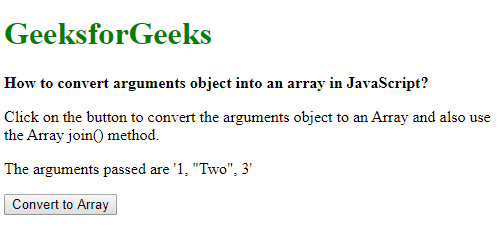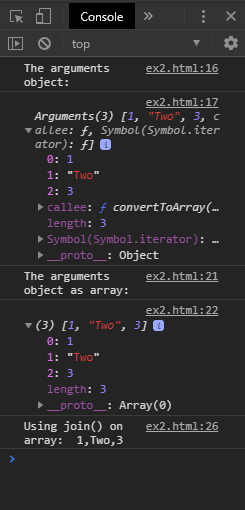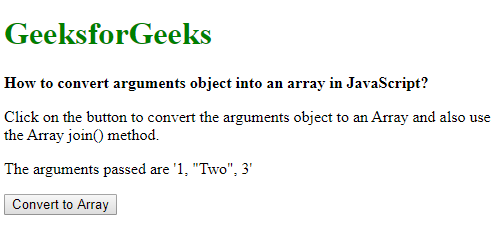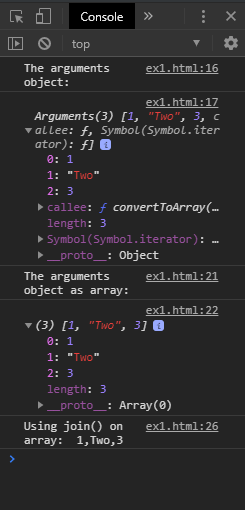# How to convert arguments object into an array in JavaScript ?

The arguments object is an array-like object that represents the arguments passed in when invoking a function. This array-like object does not have the array prototype chain, hence it cannot use any of the array methods. This object can be converted into a proper array using two approaches:

Method 1: Using the Array.from() method: The Array.from() method can be used to create a new array that is a shallow-copy of an array-like or iterable object. It contains three parameters that consist array-like object to be converted, an optional map function that can be called on every element of the array and a “this” argument that can be used with the map function.
The arguments object is passed as an argument to this method and it will return the array form of the object. This array has the array prototype chain and can be used with the various array methods.

Syntax:

`argumentsArray = Array.from(arguments)`

Example:

 ` ` `<``html``> ` `     `  `<``head``> ` `    ``<``title``> ` `        ``How to convert arguments object into ` `        ``an array in JavaScript? ` `    `` ` ` ` ` `  `<``body``> ` `    ``<``h1` `style``=``"color: green"``> ` `        ``GeeksforGeeks ` `    `` ` `     `  `    ``<``b``>  ` `        ``How to convert arguments object into ` `        ``an array in JavaScript? ` `    `` ` `     `  `    ``<``p``> ` `        ``Click on the button to convert the ` `        ``arguments object to an Array ` `        ``and also use the Array join() method. ` `    `` ` `     `  `    ``<``p``>The arguments passed are '1, "Two", 3' ` `     `  `    ``<``button` `onclick``=``"convertToArray(1, 'Two', 3)"``> ` `        ``Convert to Array ` `    `` ` `     `  `    ``<``script` `type``=``"text/javascript"``> ` `     `  `        ``function convertToArray(a, b, c) { ` `            ``console.log("The arguments object:") ` `            ``console.log(arguments); ` `         `  `            ``argumentsArray = Array.from(arguments); ` `         `  `            ``console.log("The arguments object as array:") ` `            ``console.log(argumentsArray); ` `         `  `            ``// Example of using the join() method ` `            ``joinedArray = argumentsArray.join(', '); ` `             `  `            ``console.log("Using join() on array: ", ` `                                    ``joinedArray); ` `        ``} ` `    `` ` ` ` ` `  ` `

Output:

• Display:• Console:Method 2: Using Array.prototype.slice() method: The Array.prototype.slice() method is used to return a portion of an array into a new array object. This array would be a shallow copy of the original array. This method has two optional arguments that indicate the beginning and ending index of the array. If the arguments are omitted, the indices are set to the first and last index of the array.
This method can be used to convert the arguments object by binding this method to the object. The binding is done using the call() function and passing the array-like object as an argument to it. It will return an array form of the arguments object. This array has the array prototype chain and can be used with the various array methods.

Syntax:

`argumentsArray = Array.prototype.slice.call(arguments)`

Example:

 ` ` `<``html``> ` ` `  `<``head``> ` `    ``<``title``> ` `        ``How to convert arguments object ` `        ``into an array in JavaScript? ` `    `` ` ` ` ` `  `<``body``> ` `    ``<``h1` `style``=``"color: green"``> ` `        ``GeeksforGeeks ` `    `` ` `     `  `    ``<``b``> ` `        ``How to convert arguments object into ` `        ``an array in JavaScript? ` `    `` ` `     `  `    ``<``p``> ` `        ``Click on the button to convert the ` `        ``arguments object to an Array and ` `        ``also use the Array join() method. ` `    `` ` `     `  `    ``<``p``>The arguments passed are '1, "Two", 3' ` `     `  `    ``<``button` `onclick``=``"convertToArray(1, 'Two', 3)"``> ` `        ``Convert to Array ` `    `` ` `     `  `    ``<``script` `type``=``"text/javascript"``> ` `     `  `        ``function convertToArray(a, b, c) { ` `            ``console.log("The arguments object:") ` `            ``console.log(arguments); ` `         `  `            ``argumentsArray =  ` `                ``Array.prototype.slice.call(arguments); ` `         `  `            ``console.log("The arguments object as array:") ` `            ``console.log(argumentsArray); ` `         `  `            ``// Example of using the join() method ` `            ``joinedArray =  ` `                ``argumentsArray.join(', '); ` `                 `  `            ``console.log("Using join() on array: ", ` `                                       ``joinedArray); ` `        ``} ` `    `` ` ` ` ` `  ` `

Output:

• Display:• Console:My Personal Notes arrow_drop_upIm listening

If you like GeeksforGeeks and would like to contribute, you can also write an article using contribute.geeksforgeeks.org or mail your article to contribute@geeksforgeeks.org. See your article appearing on the GeeksforGeeks main page and help other Geeks.

Please Improve this article if you find anything incorrect by clicking on the "Improve Article" button below.